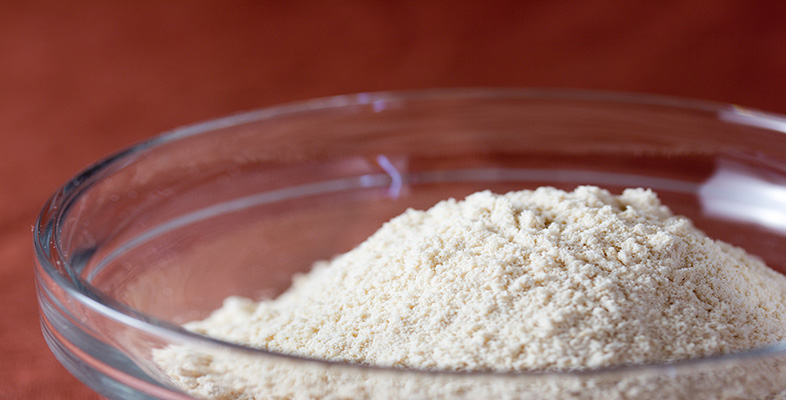Science, Maths & Technology
Free course

# Rounding and estimation

Free statement of participation on completion## Course reviews

Scientific calculators are a wonderful invention, but theyre only as good as the people who use them. If you often get an unexpected or ridiculous result when you press the enter button, this free course, Rounding and estimation, is for you. Learn how to do a calculation correctly and get the right answer every time.

## Course learning outcomes

After studying this course, you should be able to:

• round a given whole number to the nearest 10, 100, 1000 and so on
• round a decimal number to a given number of decimal places or significant figures
• use rounded numbers to find rough estimates for calculations
• use a calculator for decimal calculations involving +, −, × and ÷, giving the answer to a specified accuracy (e.g. decimal places or significant figures) and checking the answer by finding a rough estimate
• check the answers to calculations by ensuring that the correct calculation has been done, in the correct order.

First Published: 10/08/2012

Updated: 18/04/2019

Skip Rate and Review